# CFD Modelling of Pressure Drop in Double-Pipe Heat Exchanger with Turbulators Using a Porous Media Model

CFD Modelling of Pressure Drop in Double-Pipe Heat Exchanger with Turbulators Using a Porous Media Model
Department of Process Engineering and Technology of Polymer and Carbon Materials, Wrocław University of Science and Technology, Wybrzeże Wyspiańskiego 27, 50-370 Wrocław, Poland

Faculty of Chemistry, Department of Process Engineering and Technology of Polymer and Carbon Materials, Wrocław University of Science and Technology, Wybrzeże Wyspiańskiego 27, 50-370 Wrocław, Poland

Corresponding Author Email:
marcelina.gendera@pwr.edu.pl
Page:
162-170
|
DOI:
https://doi.org/10.18280/ijht.410117
14 December 2022
|
Revised:
29 January 2023
|
Accepted:
10 February 2023
|
Available online:
28 February 2023
| Citation

OPEN ACCESS

Abstract:

A major limitation of Computational Fluid Dynamics application in modelling devices with complex configuration is generating a numerical mesh that can accurately recreate the fluid domain without exceeding available computational power. To simplify the geometry of the impossible to simulate traditionally Pall rings and brush turbulators, the porous media model was implemented. In this research, we simulated the pressure drop in the double-pipe heat exchanger filled with eleven turbulators of different kinds (including: Pall rings, spiral packing, stamped sheet, brush, spring, and twisted tape). The viscous and inertial coefficients of the porous media were calculated for each turbulator and implemented in the calculations. The results were compared with the collected experimental data, which demonstrated the satisfactory accuracy of this approach. It is concluded that the porous media model performs especially well for the brush and spiral packing turbulators.

Keywords:

CFD, porous media, turbulators, pressure drop

1. Introduction

Efficient heat transfer is crucial in various industrial applications, such as food processing, refrigeration, electronics, power plants, and waste heat recovery [1-7]. Tube-in-tube heat exchangers have many economic advantages compared to other configurations and are therefore widely used in industry. Their design is relatively easy, and the maintenance and installation costs are low [8-10].

Turbulators are commonly used to passively enhance the performance of heat exchangers in a cost-effective way. Active methods to boost heat transfer require external power input, and therefore their application is limited. Examples include mechanical aids, surface and fluid vibrations, injection, or electrostatic fields. Passive methods rely on geometrical or surface modifications of the flow tube by adding devices or various inserts. The main effectiveness of turbulators lies either in reducing the available volume for the fluid, consequently increasing its velocity, or in breaking the boundary layer, where most of the heat transfer resistance is located, by directing the flow towards the walls of the exchanger. Numerous studies show that turbulators are the subject of great interest in improving heat transfer, as evident in reviews [9, 11-13]. They are easily applicable in pre-existing installations, which is a major advantage. Their downside is an increased pressure drop.

The experimental approach to design is tedious and time consuming. Because of that, numerical methods are preferred for turbulator analysis. It is crucial to develop simple and reliable models that enable the prediction and analysis of complex phenomena which occur in the turbulators.

Computational Fluid Dynamics (CFD) is a valuable tool that supports design, analysis, and engineering calculations. It is gaining more and more popularity due to the increasing necessity to quickly adapt to changing market demands, continuous advancement of new and existing technologies, and constant improvement of product quality and reduction in production costs. CFD simulations can accelerate designing and reduce the number of costly experimental tests . Hydrodynamics of the flow along with heat and mass exchange in fluids are predicted/predictable thanks to the numerical solution of complex transport equations under given conditions. Complicated CFD models still require experimental validation and further development to obtain the most reliable results.

The differential transport equations are often impossible to solve analytically, therefore they need to be changed into algebraic ones in the discretized domain. The fluid flow area is divided into small elements called computing cells, in which the extensive quantities (such as mass, energy, momentum) are balanced, which returns the field of the intense quantities (pressure, temperature). The resulting numerical mesh must faithfully reflect the real geometry of the object, be appropriately dense, while the individual elements have to maintain the suitable quality [15, 16].

This is the main obstacle in simulating turbulators. Accurate recreation of the geometry of the packed bed is practically impossible whereas generating a numerical mesh for twisted tape and spring turbulators requires enormous computing power, which often exceeds the capabilities of modern computers. A huge number of cells are formed, and sudden changes and breaks in the geometry favor the formation of elements with high skewness, which negatively affects the stability of calculations.

Implementing the porous-media approach to modelling turbulators can solve this issue. This model links the velocity of the fluid with the pressure drop in the apparatus and does not require drawing real geometry (see Section 2.2).

There are few examples in the literature of utilizing a porous media approach in simulating heat exchangers with fins in order to reduce the size of the numerical mesh and, therefore, computational power demand. Instead of drawing a complicated, yet repeatable geometry of fin-tube intercoolers, a simple overall shape of the domain is created and described using porous-media coefficients calculated from available pressure drop and velocity data. Zhang et al.  performed the experimental verification of the wavy fin air intercooler, obtaining a maximum deviation of 15% between the simulated and empirical results. Musto et al.  tested the performance of the aircraft oil intercooler (on the air side), comparing the simulation results with the manufacturer’s data, also reaching the satisfactory accuracy of the heat flux and pressure drop. A different approach involves the simulation of a part of the real geometry of the vehicle radiator, the calculation of the coefficients of the porous media from the results, and then modelling the simplified geometry by employing the porous media approach. The required mesh size was reduced by over 920 times with a 2.5% deviation from the results . It has not yet been established whether this approach will work in modelling turbulators. The aim of this research is to determine whether the porous media model can accurately represent the pressure drop in a double-pipe heat exchanger equipped with various turbulators. Pall rings, spiral packing with different configurations, brush turbulators as well as twisted tape and spring turbulators varying in pitch and diameter were tested, calculating the generated pressure drop in relation to the changing water flow.

2. Description of the Computational Model

The main governing equations for CFD simulations are conservation equations for mass (Eq. (1)) and momentum (Eq. (2)):

$-\frac{\partial \rho}{\partial t}+\nabla \cdot(\rho \vec{v})=0$        (1)

$\frac{\partial}{\partial t}(\rho \vec{v})+\nabla \cdot(\rho \vec{v} \vec{v})=-\nabla p+\nabla \cdot(\bar{\bar{\tau}})+\rho \vec{g}+\vec{F}$        (2)

$\bar{\bar{\tau}}=\mu\left[\left(\nabla \vec{v}+\nabla \vec{v}^T\right)-\frac{2}{3} \nabla \cdot \nabla \vec{v} I\right]$        (3)

2.1 Turbulence models

The exact solution of the governing Navier-Stokes equations for turbulent flows is possible only for simple single-phase cases; it requires high computing power and is very time-consuming. Therefore, precise though it is, the Direct Numerical Simulation (DNS) method is rarely used.

The most economical are Reynolds Averaged Navier-Stokes (RANS) methods, which average vector and scalar quantities over time, introducing time mean values and value deviations , described by Eq. (4):

$v_i=\bar{v}_i+v_i^{\prime}$          (4)

They require the introduction of additional equations describing the turbulent stress tensor:

$\frac{\partial \rho}{\partial t}+\frac{\partial \rho}{\partial x_i}\left(\rho \bar{v}_l\right)=0$           (5)

$\left(\rho \bar{v}_l\right)+\frac{\partial \rho}{\partial x_j}\left(\rho \bar{v}_l \bar{v}_J\right)$ $=-\frac{\partial \bar{p}}{\partial x_i}+\frac{\partial}{\partial x_j}\left[\mu\left(\frac{\partial \bar{v}_l}{\partial x_j}+\frac{\partial \bar{v}_J}{\partial x_i}-\frac{2}{3} \delta_{i j} \frac{\partial \overline{v_k}}{\partial x_k}\right)\right]$$+\frac{\partial}{\partial x_j}\left(-\rho \overline{v_l^{\prime} v_J^{\prime}}\right)+\rho g_i+S_i (6) Models based on the Boussinesq hypothesis  introduce the concept of turbulent viscosity to describe the turbulent stress tensor, defined as follows: -\rho \overline{\bar{v}_l^{\prime} v_J^{\prime}}=\mu_t\left(\frac{\partial \bar{v}_l}{\partial x_j}+\frac{\partial \bar{v}_j}{\partial x_i}\right)-\frac{2}{3}\left(\rho k+\mu_t \frac{\partial v_k}{\partial x_k}\right) \delta_{i j} (7) The two most important Boussinesq models are k-\varepsilon and k-w. In these models two other transport equations are added: one for the specific kinetic energy of turbulence k (which is the sum of the average kinetic energy and the instantaneous kinetic energy value, describing the turbulence fluctuations), and one for either the magnitude of the kinetic energy dissipation \varepsilon, or the specific dissipation rate w. The turbulent viscosity is computed as a function of k and \varepsilon, or k and w. The standard k-\varepsilon model  is regarded as relatively easy to use and it leads to stable computations but its disadvantages are the simplified description of energy dissipation and the fact that it only works for flows with developed turbulence, therefore its applicability for specific cases always needs to be verified. The k-w model, developed by Wilcox  possesses similar properties to k-\varepsilon, with better accuracy in lower Reynolds numbers. It is, however, highly sensitive to changes in the boundary conditions at the inlet with free flow. This problem is solved by its modification, SST (Shear Stress Transport) k-w, proposed by Menter . It is a combination of the k-\varepsilon model, which is used in the fluid core, while k-w is applied in the boundary layer. Governing equations and standard parameters for this model are as follow: \frac{\partial}{\partial t}(\rho k)+\frac{\partial}{\partial x_i}\left(\rho k \bar{v}_i\right)$$=\frac{\partial}{\partial x_j}\left(\Gamma_k \frac{\partial k}{\partial x_j}\right)+G_k-Y_k+S_k+G_b$          (8)

$\frac{\partial}{\partial t}(\rho \omega)+\frac{\partial}{\partial x_i}\left(\rho \omega \bar{v}_i\right)$$=\frac{\partial}{\partial x_j}\left(\Gamma_\omega \frac{\partial \omega}{\partial x_j}\right)+G_\omega-Y_\omega+D_\omega+S_\omega+G_{\omega b} (9) Gk and Gw are terms responsible for the production of k and w. \Gamma_k and \Gamma_\omega represent the effective diffusivity. Yk and Yw describe the dissipation of k and w due to turbulence. Dw is a cross-diffusion term. Sk and Sw are user-defined source terms. Gb and Gbw account for buoyancy. \Gamma_k=\mu+\frac{\mu_t}{\sigma_k} (10) \Gamma_\omega=\mu+\frac{\mu_t}{\sigma_\omega} (11) \sigma_k=\frac{1}{\frac{F_1}{\sigma_{k, 1}}+\frac{1-F_1}{\sigma_{k, 2}}} (12) \sigma_\omega=\frac{1}{\frac{F_1}{\sigma_{\omega, 1}}+\frac{1-F_1}{\sigma_{\omega, 2}}} (13) where, \quad \sigma_{k, 1}=1.176 ; \quad \sigma_{\omega, 1}=2.0 ; \quad \sigma_{k, 2}=1.0 ; \quad \sigma_{\omega, 2}=1.168 ; \quad F_1= \tanh \left(\Phi_1^4\right) ; \quad \Phi_1=\min \left[\max \left(2 \frac{\sqrt{k}}{0.09 \omega y}, \frac{500 \mu}{\rho y^2 \omega}\right), \frac{4 \rho k}{\sigma_{\omega, 2} D_\omega^{+} y^2}\right]; D_\omega^{+}=\max \left[2 \rho \frac{1}{\sigma_{\omega, 2}} \frac{1}{\omega} \frac{\partial k}{\partial x_j} \frac{\partial \omega}{\partial x_j}, 10^{-10}\right] ; D_\omega^{+}is the positive portion of the cross-diffuse term; y is the distance to the next surface. \mu_t=\frac{\rho k}{\omega} \frac{1}{\max \left[\frac{1}{\alpha^*}, \frac{S F_2}{a_1 \omega}\right]} (14) where, F_2=\tanh \left(\Phi_2^2\right) \Phi_2=\max \left[2 \frac{\sqrt{k}}{0.09 \omega y}, \frac{500 \mu}{\rho y^2 \omega}\right]; a1=0.31. \alpha^*=\alpha_{\infty}^*\left(\frac{\alpha_0^*+\frac{R e_t}{R_k}}{1+\frac{R e_t}{R_k}}\right) (15) where, R e_t=\frac{\rho k}{\mu \omega} ; R_k=6 ; \alpha_0^*=\frac{\beta_i}{3} ; \beta_i=0.072. For high-Reynolds number \alpha^*=\alpha_{\infty}^*=1. G_k=-\rho \overline{v_i^{\prime} v_j^{\prime}} \frac{\partial \bar{v}_j}{\partial x_i}=\mu_t S^2 (16) S \equiv \sqrt{2 S_{i j} S_{i j}} (17) G_\omega=\frac{\alpha \alpha^*}{v_t} G_k (18) \alpha=\alpha_{\infty}\left(\frac{\alpha_0+\frac{R e_t}{R_\omega}}{1+\frac{R e_t}{R_\omega}}\right) (19) where, Rω=2.95; \alpha_0=\frac{1}{9}. \alpha_{\infty}=F_1 \alpha_{\infty, 1}+\left(1-F_1\right) \alpha_{\infty, 2} (20) where, \alpha_{\infty, 1}=\frac{\beta_{i, 1}}{\beta_{\infty}^*}-\frac{\kappa^2}{\sigma_{\omega, 1} \sqrt{\beta_{\infty}^*}} ; \alpha_{\infty, 2}=\frac{\beta_{i, 2}}{\beta_{\infty}^*}-\frac{\kappa^2}{\sigma_{\omega, 2} \sqrt{\beta_{\infty}^*}} ; k=0.41; βi,1=0.075; βi,2=0.0828. Y_k=\rho \beta^* k \omega (21) where, \beta^*=\beta_i^*\left[1+\zeta^* F\left(M_t\right)\right] ; \beta_i^*=\beta_{\infty}^*\left(\frac{\frac{4}{15}+\left(\frac{R e_t}{R_\beta}\right)^4}{1+\left(\frac{R e_t}{R_\beta}\right)^4}\right) ; \zeta^*= 1.5 ; R_\beta=8 ; \beta_{\infty}^*=0.09. F(Mt) is the compressibility function. In the incompressible flows \beta^*=\beta_i^*. In the high-Reynolds number form \beta_i^*=\beta_{\infty}^*. Y_\omega=\rho \beta \omega^2 (22) \beta=\beta_i\left[1-\frac{\beta_i^*}{\beta_i} \zeta^* F\left(M_t\right)\right] (23) \beta_i=F_1 \beta_{i, 1}+\left(1-F_1\right) \beta_{i, 2} (24) G_{\omega b}=\frac{\omega}{k}\left[(1+\alpha) C_{3 \varepsilon} G_b-G_b\right] (25) The SST k-w model is able to accurately represent both laminar and turbulent flows . Menter et al.  developed the Intermittency Transition Model, specifically to describe the laminar-to-turbulent transition. The model is later coupled with SST k-w. Added governing equations are as follow: \frac{\partial(\rho \gamma)}{\partial t}+\frac{\partial\left(\rho U_j \gamma\right)}{\partial x_j}=P_\gamma-E_\gamma+\frac{\partial}{\partial x_j}\left[\left(\mu+\frac{\mu_t}{\sigma_\gamma}\right) \frac{\partial \gamma}{\partial x_j}\right] (26) P_\gamma=F_{\text {length }} \rho S \gamma(1-\gamma) F_{\text {onset }} (27) where, S is a strain rate magnitude and Flength=100. E_\gamma=c_{a 2} \rho \Omega_\gamma F_{t u r b}\left(c_{e 2} \gamma-1\right) (28) where, c_{a 2}=0.06 ; c_{e 2}=50 ; \Omega_\gamma=1.0 ; F_{\text {onset }}=\max \left(F_{\text {onset2 }}-F_{\text {onset3 }}, 0\right); F_{t u r b}=e^{-\left(\frac{R_T}{2}\right)^4} ; R_T=\frac{\rho k}{\mu \omega} ; R e_V=\frac{\rho d_w^2 S}{\mu}. To calculate the critical momentum thickness Reynolds number, the freestream turbulence intensity and pressure gradient are approximated locally: R e_{\theta c}=f\left(T u_L, \lambda_{\theta L}\right) R e_{\theta c}\left(T u_L, \lambda_{\theta L}\right)=C_{T U 1} +C_{T U 2} \exp \left[-C_{T U 3} T u_L F_{P G}\left(\lambda_{\theta L}\right)\right] T u_L=\min \left(100 \frac{\sqrt{\frac{2 k}{3}}}{\omega d_w}, 100\right) (29) \lambda_{\theta L}=-7.57 \cdot 10^{-3} \frac{d V}{d y} \frac{d_w^2}{v}+0.0128 To ensure numerical robustness, \lambda_{\theta L} is bounded: \lambda_{\theta L}=\min \left(\max \left(\lambda_{\theta L},-1.0\right), 1.0\right) (30) F_{P G}\left(\lambda_{\theta L}\right)$$=\left\{\begin{array}{r}\min \left(1+C_{P G 1} \lambda_{\theta L}, C_{P G 1}^{l i m}\right), \lambda_{\theta L} \geq 0 \\ \min \left(1+C_{P G 2} \lambda_{\theta L}+C_{P G 3} \min \left[\lambda_{\theta L}+0.0681,0\right], C_{P G 2}^{l i m}\right), \lambda_{\theta L}<0\end{array}\right.$           (31)

$F_{P G}=\max \left(F_{P G}, 0\right)$           (32)

Model constants: $\quad C_{T U 1}=100.0 ; \quad C_{T U 2}=1000.0 ; \quad C_{T U 3}=1.0 ;$ $C_{P G 1}=14.68 ; C_{P G 2}=-7.34 ; C_{P G 3}=0.0 ; C_{P G 1}^{l i m}=1.5 ; C_{P G 2}^{l i m}=3.0$.

The model was established for the external flows, to adjust it for internal flows Abraham et al.  proposed the change to constant values as follows: CTU1=75.0; CTU3=1.37; β*=0.092.

All described models are suitable for modelling transitional flow, that occurred during the experimental research (see Section 3).

2.2 Porous media model

The porous media model is based on the Darcy-Forchheimer equation, which describes fluid flow through porous media that differs from Darcy's law. At a high Reynolds number, the inertial forces grow more prominent, and the relationship between pressure gradient and seepage velocity becomes non-linear. When those forces are of higher order than viscous forces, non-Darcy flow can be described with the empirical Forchheimer equation :

$-\nabla P=\frac{\mu}{\alpha_p} v+\beta_p \rho v^2$     (33)

The inertial $\left(\beta_p\right)$ and viscous (αp-1) coefficients of the porous media model need to be calculated from experimental data.

The porous media model in ANSYS Fluent adds the momentum sink to the governing momentum equations. The momentum source term consists of a viscous loss (Darcy) term and an inertial loss term, as presented in the following equation :

$S_i=-\left(\sum_{j=1}^3 D_{i j} \mu v_j+\sum_{j=1}^3 C_{i j} \frac{1}{2} \rho|v| v_j\right)$         (34)

By default, the built-in model uses superficial velocity, so the porous media coefficients from the experimental data need to be calculated accordingly. The effect of the porous zone on the turbulence field is only approximated. In the default approach, the solid medium has no effect on turbulence generation, which is a reasonable assumption if its permeability is large, i.e., typical of turbulators.

3. Modelled Apparatus and Experimental Data

To obtain the experimental data necessary to calculate the coefficients of the porous medium and to validate the computational results, static pressure drops were measured in a double-piped brass heat exchanger, whose inner tube was filled with different turbulators [28-32]. The schematic diagram of the tested device is presented in Figure 1.

## 1.png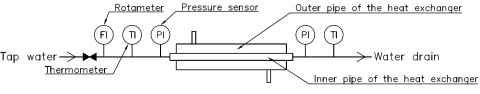Figure 1. Schematic diagram of the modelled tube-in-tube heat exchanger

The apparatus is 585 mm long, the diameter of the inner tube is 11 mm with a 2.5 mm thick wall, and the diameter of the outer tube is 28 mm with a 2 mm thick wall. The exchanger is wrapped with the heat-insulating mat. The inner tube of the exchanger was filled with various turbulators, which are presented in Figure 2. Pall rings and stamped sheet turbulators affect the turbulence mainly by presenting an obstacle to the fluid, while twisted tape and spring turbulators break the boundary layer. Spiral packing and brush-ramrod turbulator (and their modifications adding the rings between segments) likely combine both effects.

## 2.png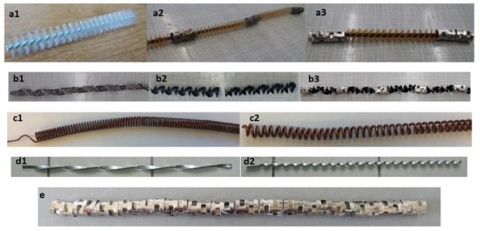Figure 2. Various types of turbulators used in the simulations:

a1) brush turbulator a2) brush turbulator with Białecki rings a3) brush turbulator with Pall rings 

b1) stamped sheet turbulator b2) spiral packing turbulator b3) spiral packing turbulator with Pall rings 

c1) c2) spring turbulators differing in pitch and diameter 

d1) d2) twisted tape turbulators differing in pitch  e) Pall rings 

Pressure drops were measured in relation to the change in the flow rate of cold water flowing through the inner pipe. The mean inlet temperature of the water was 13°C (density: 999,3 kg/m3, dynamic viscosity: 1.20×10-3 Pa·s). Its mass flow rate was changed in the range of 0.022-0.078 kg/s, resulting in Reynolds numbers (calculated for the superficial velocity) between 2122 and 7522.

The results of the pressure drops for each turbulator are presented in Figure 3.

The figure clearly shows that the twisted-tape turbulators generate the lowest pressure drop (between 2 to 6 times increase compared to the empty pipe). The more twists per unit of length, the higher the pressure drop. The higher number of coils also increases the pressure drop in spring turbulators, which – compared to empty pipe – increases the pressure drop by 10 to 13 times. The stamped sheet turbulator increases the pressure drop around 15 times. The Pall rings by themselves create the moderate pressure drop (about 22-26 times compared to the empty pipe), but their addition to spiral packing raises the pressure drop compared to unmodified spiral packing. The reverse tendency is observed for brush turbulators. Brush turbulators generate the highest pressure drop (more than 75 times greater than in the empty pipe) of all the discussed turbulators. The addition of Białecki rings does not affect the pressure drop much, they increase the pressure drop by around 2.6% compared to the unmodified brush turbulator. The lowest pressure drop is generated by turbulators that mainly affect the boundary layer and do not take much volume.

According to the experimental data, the inertial and viscous coefficients of the Darcy-Forchheimer equation were approximated by applying scripts written by authors, based on the least-squares method. All of the obtained curves showed a good data fit, with the values of R-squared above 99%. The coefficients for each turbulator are presented in Table 1. It can be observed that the high pressure drop is related to the large values of the viscous coefficient.

## 3.png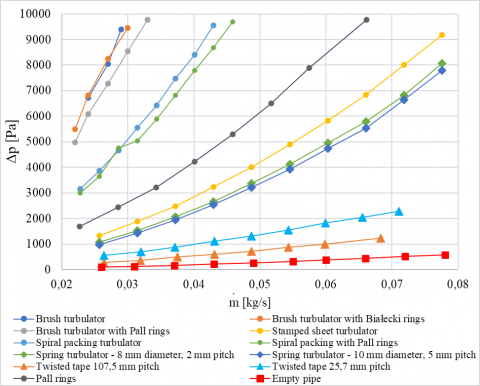Figure 3. Static pressure drop in relation to mass flow rate for examined turbulators [28-32]

Table 1. Porous-media coefficients for turbulators

 Turbulator type Viscous coefficient Inertial coefficient R-squared,% Brush Turbulator 8.31 × 106 277 99.87 Brush Turbulator with Białecki Rings 1.05 × 107 252 99.76 Brush Turbulator with Pall Rings 9.64 × 106 219 99.74 Stamped Sheet 2.89 × 106 39.4 99.99 Spiral Packing 6.38 × 106 128 99.98 Spiral Packing with Pall Rings 7.75 × 106 108 99.86 Spring Turbulator – 8 mm diameter, 2 mm pitch 1.55 × 106 36.6 99.97 Spring Turbulator – 10 mm diameter, 5 mm pitch 1.06 × 106 36.6 99.96 Twisted Tape – 107.5 mm pitch 8.69 × 105 5.48 99.94 Twisted Tape – 25.7 mm pitch 1.86 × 106 8.14 99.92 Pall Rings 5.13 × 106 53.1 99.95
4. Selection of Model Parameters

The CFD simulations were performed using the ANSYS software package, particularly the Fluent solver. Only the inner tube of the heat exchanger was modelled since this is where the turbulators were located and the pressure was measured.

4.1 Selection of a numerical mesh

The first stage of the simulation is to create a numerical mesh. The mesh independence study ensures that mesh quality does not affect results. Examples of generated meshes and their properties are shown in Table 2. Polyhedral meshes allow good geometry fit and high quality while significantly reducing the number of cells. Since a high gradient of velocity values was expected on the walls, each time the boundary layer of ten cells was added. To test the mesh two turbulators were chosen for the calculations – one representing turbulators affecting mainly the boundary layer, and one taking up the whole volume of the pipe. Simulations were performed for each mesh by the SST k-w turbulence model. The relative errors of the static pressure drop were calculated for each data point.

$\delta_p=\frac{\Delta p_{c a l c}-\Delta p_{e m p}}{\Delta p_{e m p}} \cdot 100 \%$     (35)

The results are shown in Figure 4. The medium-size mesh, selected due to the limited computational power available, was chosen to find out the balance between the calculation time and accuracy of the results. The average relative error drops only by less than 1 percentage point for the larger mesh, while the calculations lasted over 4 times longer.

Table 2. Properties of the generated meshes

Numerical mesh

## 2.1.png## 2.2.png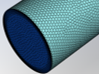## 2.3.pngNumber of cells

127 thousand

1.52 million

5.38 million

Maximum size of a surface cell

1.46 mm

0.46 mm

0.26 mm

Max Skewness

0.36

0.47

0.55

Average Skewness

0.02

0.01

0.01

Average relative error for the brush turbulator with Pall Rings

10.7%

9.1%

9.0%

Average Relative Error for the spring turbulator – 8 mm diameter, 2 mm pitch

21.9%

19.4%

18.6%

## 4.pngFigure 4. Static pressure drop for spring turbulator ϕ8 mm (ST) and brush turbulator with Pall rings (BT) for various mesh density

4.2 Selection of a turbulence model

With the mesh being selected, three relevant turbulence models offered by ANSYS Fluent, suitable for simulating transitional flows (see Section 2.1), were compared. The average relative errors along with their standard deviations for each model are presented in Table 3. The standard deviation indicates how close individual errors are to the reported average.

It can be observed that altering the constants in the intermittency model does not affect the results at all in the discussed flow regime. Furthermore, in our case, the standard SST k-w model performs just as well, while reducing computational effort (no need to solve additional equations). This model is recommended by ANSYS. It was chosen as the most economical option for future calculations, given that the calculation time was shorter, and the difference in the results negligible.

Table 3. Average relative error and standard deviation for each turbulence model for the numerical mesh of 1.52 million cells for different turbulators

 Spring turbulator – 8 mm diameter Brush turbulator with Pall rings Turbulence Model 1 2 3 1 2 3 Average Relative Error,% 19.4 18.8 18.8 9.13 9.12 9.12 Standard deviation,% 2.29 2.14 2.14 2.69 2.70 2.70

Turbulence models: 1 - SST k-w; 2 - Intermittency model; 3 - Intermittency model with changed constants

4.3 Simulations of the pressure drop for turbulators

The coefficients presented in Table 1 were implemented in the porous medium model and simulations were performed. The results of the average relative error and standard deviation for each turbulator are presented in Table 4.

Table 4. Average relative error and standard deviation for each turbulator for the SST k-omega turbulence model

 Turbulator type Average relative error,% Standard deviation,% Brush Turbulator 9.7 1.5 Brush Turbulator with Białecki Rings 8.8 2.5 Brush Turbulator with Pall Rings 9.1 2.7 Stamped Sheet 17.3 2.3 Spiral Packing 10.9 0.9 Spiral Packing with Pall Rings 10.6 3.2 Spring Turbulator – 8 mm diameter, 2 mm pitch 19.4 2.3 Spring Turbulator – 10 mm diameter, 5 mm pitch 20.4 3.1 Twisted Tape – 107.5 mm pitch 64.3 4.0 Twisted Tape – 25.7 mm pitch 40.5 2.5 Pall Rings 15.1 2.2

The degree to which the porous medium model approximates the pressure drop in the turbulators can be correlated with the values of the inertial coefficients presented in Table 1. The greater the influence of inertial forces, the more the turbulator resembles the porous media, and better accuracy is achieved. Twisted tape turbulators have the lowest inertial coefficients. They do not block the fluid flow in the way a porous media would; hence the low accuracy. Stamped sheet and spring turbulators have similar relative errors and medium inertial coefficients. Pall rings could resemble porous media of medium porosity and the relative error corresponds to that. Brush turbulators occupy most of the space, have the highest inertial coefficients, and the lowest errors. It can be concluded that turbulators that mainly affect turbulence by creating an obstacle for the flow at the fluid core are better described by the porous media model than turbulators that act on the boundary layer.

5. Comparison with the Real-Geometry Approach

The spring turbulators are approximated quite well by the porous media model, and their real geometry is easy to draw; therefore, a comparison between the two approaches is important. The 8 mm diameter spring turbulator with a 2 mm pitch was chosen for simulation and its model is presented in Figure 5. The outlet of the simulated device had to be moved downstream because of the reversed flow occurring at the end of the turbulator. The created mesh (Figure 6) consists of over 16.09 million computational cells with an average skewness of 0.02 (maximum skewness 0.80). It is of poorer quality compared to the mesh fit for the porous media approach (See Table 2). Furthermore, the full geometry of the spring is still only a simplification of the real-life assembly. In the actual device the spring was lying at the bottom of the pipe rather than in its axis, but the accurate mesh for this case was impossible to generate.

## 5.pngFigure 5. Geometry model of the spring turbulator with 8 mm diameter and 2 mm pitch

## 6.png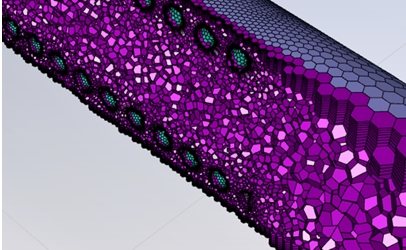Figure 6. Polyhedral numerical mesh for the spring turbulator

## 7.png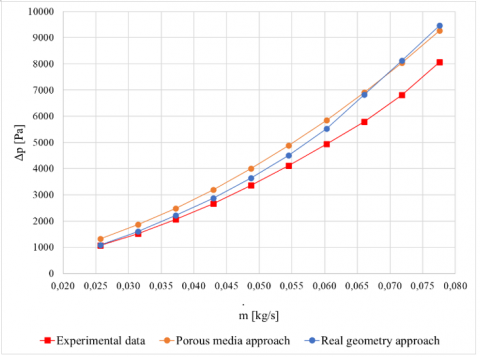Figure 7. Comparison of the real geometry and the porous media approach for the spring turbulator with 8 mm diameter and 2 mm pitch

The results (Figure 7) obtained with the real geometry approach are better (the average relative error is 11% compared to 19% for the porous media approach), but the calculation time was more than 10 times longer. For a higher mass flow rate, the porous media approach gives better results. The apparent crossing of the data could be explained by numerical errors due to the quality of the mesh or the imperfect recreation of the real geometry. It would need to be further investigated for even higher Reynolds numbers. The comparatively small differences between both solutions show that the porous media approach in modelling turbulators is an interesting subject that could potentially aid CFD analysis in the future.

6. Conclusions

This research provided a baseline understanding of the problem of simulating turbulators using the porous media model, which has not been discussed so far. It is a good starting point for future exploration.

The porous media model works best with turbulators described by high inertial coefficients. Their common trait is that they all occupy a high percentage of the cross-section of the pipe and cause the highest pressure drop.

The porous media model cannot be used to describe twisted tape turbulators. Their effectiveness lies mainly in directing the flow towards the walls of the device and breaking the boundary layer, which is not modelled properly.

Modelling the real geometry of the turbulators is very time-consuming, and the results are highly dependent on the quality of the mesh; therefore, it would be advisable to simplify the process.

It is worth noting that the porous media model returns a higher value of the pressure drop than in reality, which is fine for engineering applications, since continuous flow must always be ensured while designing an installation, and it is better to overestimate than to underestimate the pressure drop.

Modelling the pressure drop in turbulators using the porous media approach gives promising results and should be further developed. The next stage of the research would be to introduce heat exchange into the simulation.

Nomenclature
 C3ε buoyancy-related coefficient in k-ε models Cx model constant Cij porous medium matrix describing inertial resistance, m-1 Dij porous medium matric describing viscous resistance, m-2 d diameter, m dw wall distance, m $\vec{F}$ external body forces and model-dependent source terms, N Gb generation of turbulence kinetic energy due to buoyancy, kg∙m-1∙s-3 Gk generation of turbulence kinetic energy due to mean velocity gradients, kg∙m-1∙s-3 $\vec{g}$ gravitational acceleration vector, m-1∙s2 k turbulence kinetic energy, J∙kg-1 I unit tensor p static pressure, Pa Pr Prandtl number Re Reynolds number $R e_{\theta c}$ critical momentum thickness Reynolds number Si momentum source term in the porous media model, N∙m-3 Sij mean-strain-rate tensor, s-1 Sk user-defined source of turbulence kinetic energy, kg∙m-1∙s-3 Sε user-defined source of turbulence kinetic energy dissipation rate, kg∙m-1∙s-4 t time, s T temperature, K $T u$ freestream turbulence intensity,% $\vec{v}$ velocity vector, m∙s-1 $\bar{v}$ mean (Reynolds-averaged) velocity, m∙s-1 $\boldsymbol{v}$ velocity, instantaneous velocity, m∙s-1 $v^{\prime}$ fluctuating part of velocity, m∙s-1 $y$ distance, m Greek symbols α inverse turbulent Prandtl number αp permeability of the porous medium, m2 β thermal expansion coefficient, K-1 βp inertial Forchheimer coefficient (non-Darcy coefficient), m-1 $\gamma$ intermittency $\delta_{\mathrm{ij}}$ Kronecker delta ε turbulence kinetic energy dissipation rate, m2×s-3 μ dynamic viscosity, Pa×s $\mu_{e f f}$ effective viscosity, Pa×s $\mu_t$ turbulent viscosity, Pa×s ρ density, kg×m-3 $\sigma_k$ turbulent Prandtl number for the turbulent kinetic energy $\sigma_{\varepsilon}$ turbulent Prandtl number for the turbulent kinetic energy dissipation $\bar{\bar{\tau}}$ stress tensor, Pa $\Gamma$ effective diffusivity, m2×s-1 $\omega$ specific dissipation rate, s-1 $I$ mean rotation rate tensor, s-1 $\Omega$ magnitude of the absolute vorticity rate, s-1 Subscripts i the i-th element of a vector ij i-th row and j-th column element of a tensor k the k-th element of a vector
References

 Guo, Z., Cheng, L., Cao, H., Zhang, H., Huang, X., Min, J. (2021). Heat transfer enhancement: A brief review of literature in 2020 and prospects. Heat Transfer Research, 52: 65-92. https://doi.org/10.1615/HEATTRANSRES.2021038770

 Alam, T., Kim, M.H. (2018). A comprehensive review on single phase heat transfer enhancement techniques in heat exchanger applications. Renewable and Sustainable Energy Reviews, 81: 813-839. https://doi.org/10.1016/J.RSER.2017.08.060

 Rashidi, S., Kashefi, M.H., Kim, K.C., Samimi-Abianeh, O. (2019). Potentials of porous materials for energy management in heat exchangers – A comprehensive review. Applied Energy, 243: 206-232. https://doi.org/10.1016/J.APENERGY.2019.03.200

 Niknam, S.A., Mortazavi, M., Li, D. (2021). Additively manufactured heat exchangers: A review on opportunities and challenges. International Journal of Advanced Manufacturing Technology, 112: 601-618. https://doi.org/10.1007/S00170-020-06372-W/TABLES/2

 Ramesh, K.N., Sharma, T.K., Rao, G.A.P. (2020). Latest advancements in heat transfer enhancement in the micro-channel heat sinks: A review. Archives of Computational Methods in Engineering, 28: 3135-3165. https://doi.org/10.1007/S11831-020-09495-1

 Ghalandari, M., Irandoost Shahrestani, M., Maleki, A., Safdari Shadloo, M., el Haj Assad, M. (2021). Applications of intelligent methods in various types of heat exchangers: A review. Journal of Thermal Analysis and Calorimetry, 145: 1837-1848. https://doi.org/10.1007/S10973-020-10425-3/TABLES/1

 Françolle De Almeida, C., Saget, M., Delaplace, G., Jimenez, M., Fierro, V., Celzard, A. (2021). Innovative fouling-resistant materials for industrial heat exchangers: A review. Reviews in Chemical Engineering. https://doi.org/10.1515/REVCE-2020-0094/ASSET/GRAPHIC/J_REVCE-2020-0094_FIG_010.JPG

 Hangi, M., Rahbari, A., Lipiński, W. (2021). Design improvement of compact double-pipe heat exchangers equipped with tube-side helical insert and annulus-side helical strip: Hydrothermal and exergy analyses. Applied Thermal Engineering, 190: 116805. https://doi.org/10.1016/J.APPLTHERMALENG.2021.116805

 Li, H., Wang, Y., Han, Y., Li, W., Yang, L., Guo, J., Liu, Y.D., Zhang, J.L., Zhang, M.Q., Jiang, F. (2021). A comprehensive review of heat transfer enhancement and flow characteristics in the concentric pipe heat exchanger. Powder Technology, 397: 117037. https://doi.org/10.1016/J.POWTEC.2021.117037

 Omidi, M., Farhadi, M., Jafari, M. (2017). A comprehensive review on double pipe heat exchangers. Applied Thermal Engineering, 110: 1075-1090. https://doi.org/10.1016/J.APPLTHERMALENG.2016.09.027

 Suri, A.R.S., Kumar, A., Maithani, R. (2017). Convective heat transfer enhancement techniques of heat exchanger tubes: A review. International Journal of Ambient Energy. 39: 649-670. https://doi.org/10.1080/01430750.2017.1324816

 Sheikholeslami, M., Gorji-Bandpy, M., Ganji, D.D. (2015). Review of heat transfer enhancement methods: Focus on passive methods using swirl flow devices. Renewable and Sustainable Energy Reviews, 49: 444-469. https://doi.org/10.1016/J.RSER.2015.04.113

 Rajesh Babu, C., Kumar, P., Sukanta, R., Rajamohan, G. (2022). A comprehensive review on compound heat transfer enhancement using passive techniques in a heat exchanger. Materials Today: Proceedings, 54: 428-436. https://doi.org/10.1016/J.MATPR.2021.09.541

 Aslam Bhutta, M.M., Hayat, N., Bashir, M.H., Khan, A.R., Ahmad, K.N., Khan, S. (2012). CFD applications in various heat exchangers design: A review. Applied Thermal Engineering, 32: 1-12. https://doi.org/10.1016/J.APPLTHERMALENG.2011.09.001

 Blazek, J. (2005). Computational fluid dynamics: Principles and applications. Elsevier Ltd. https://doi.org/10.1016/B978-0-08-044506-9.X5000-0

 Kajishima, T., Taira, K. (2017). Computational Fluid Dynamics. 1st ed. Springer International Publishing, Cham. https://doi.org/10.1007/978-3-319-45304-0

 Zhang, Q., Qin, S., Ma, R. (2016). Simulation and experimental investigation of the wavy fin-and-tube intercooler. Case Studies in Thermal Engineering, 8: 32-40. https://doi.org/10.1016/j.csite.2016.04.003

 Musto, M., Bianco, N., Rotondo, G., Toscano, F., Pezzella, G. (2016). A simplified methodology to simulate a heat exchanger in an aircraft’s oil cooler by means of a Porous Media model. Applied Thermal Engineering, 94: 836-845. https://doi.org/10.1016/j.applthermaleng.2015.10.147

 Çetin, B., Güler, K.G., Aksel, M.H. (2017). Computational modeling of vehicle radiators using porous medium approach. Heat Exchangers - Design, Experiment and Simulation. https://doi.org/10.5772/66281

 Wilcox, D.C. (1998). Turbulence Modeling for CFD. DCW Industries.

 Hinze, J.O. (1975). TURBULENCE. 2nd edition. McGraw-Hill.

 Launder, B.E., Spalding, D.B. (1972). Lectures in mathematical models of turbulence. Academic Press.

 Menter, F.R. (1994). Two-equation eddy-viscosity turbulence models for engineering applications. AIAA JOURNAL, 32. https://doi.org/10.2514/3.12149

 Gorman, J., Bhattacharyya, S., Cheng, L., Abraham, J. (2021). Turbulence models commonly used in CFD. Computational Fluid Dynamics [Working Title], IntechOpen. https://doi.org/10.5772/INTECHOPEN.99784

 Menter, F.R., Smirnov, P.E., Liu, T., Avancha, R. (2015). A one-equation local correlation-based transition model. Flow, Turbulence and Combustion, 95: 583-619. https://doi.org/10.1007/S10494-015-9622-4

 Abraham, J.P., Sparrow, E.M., Gorman, J.M., Zhao, Y., Minkowycz, W.J. (2019). Application of an intermittency model for laminar, transitional, and turbulent internal flows. Journal of Fluids Engineering, 141. https://doi.org/10.1115/1.4042664

 Zolotukhin, A.B., Gayubov, A.T. (2021). Semi-analytical approach to modeling forchheimer flow in porous media at meso- and macroscales. Transport in Porous Media, 136: 715-741. https://doi.org/10.1007/S11242-020-01528-4/FIGURES/10

 Kaczorowska, W. (2021). Use of turbulizers in heat exchange [Master’s Thesis]. Wrocław University of Science and Technology.

 Brzezińska, A. (2020). Application of turbulizers for heat transfer improvement [Master’s Thesis]. Wrocław University of Science and Technology.

 Wiśniewska, J. (2013). Determination of heat transfer conditions in double pipe heat exchange equipped with turbulizers [Master’s Thesis]. Wrocław University of Science and Technology.

 Kunce, K. (2019). Heat transfer and pressure drop in the exchanger equipped with spiral turbulator [Master’s Thesis]. Wrocław University of Science and Technology.

 Lach, J. (2018). Application of Pall rings in the process of heat transfer [Master’s Thesis]. Wrocław University of Science and Technology.